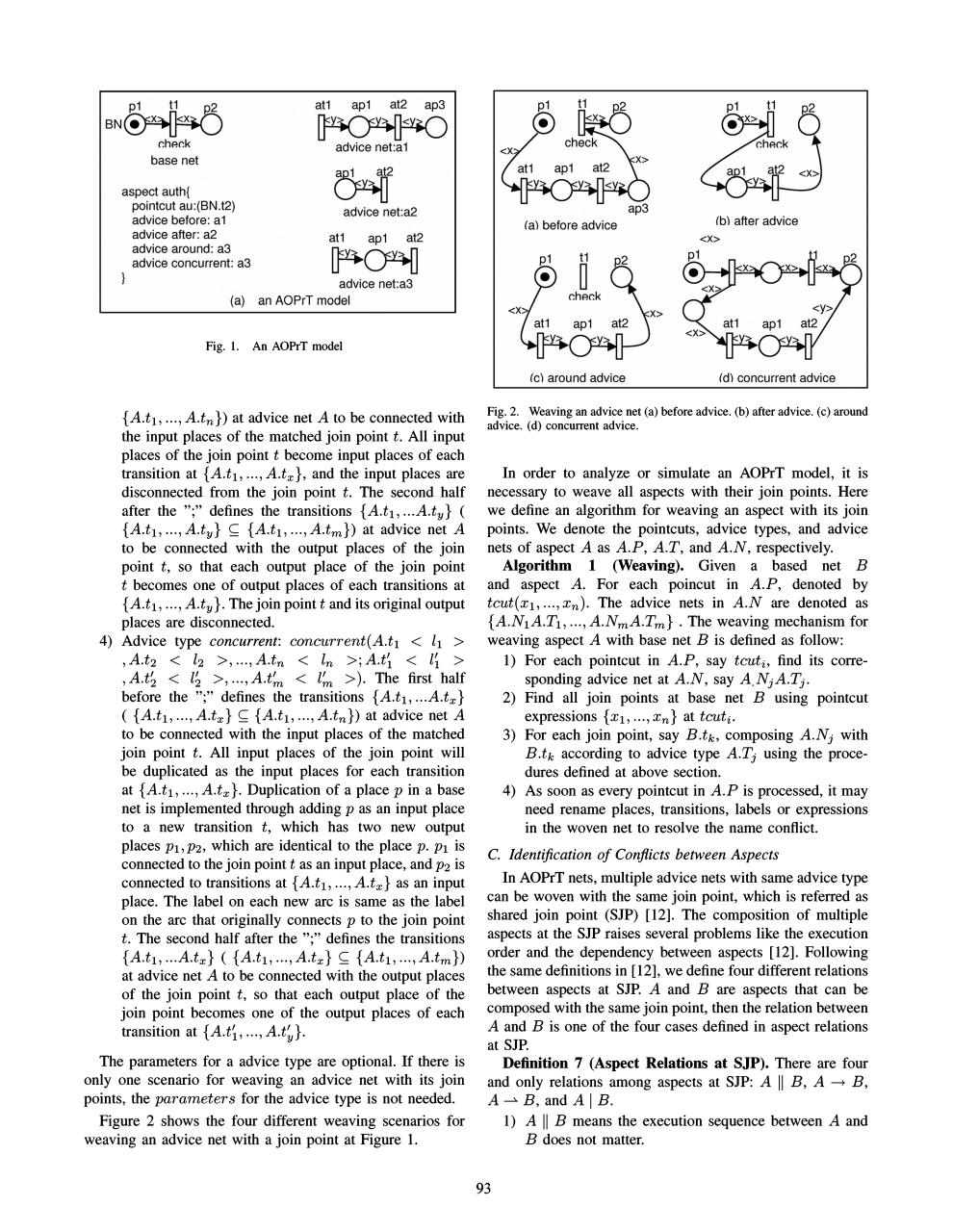# 05203910.pdfPage 1 2 3 4 5 6 7

#### Text preview

~hfL,,~

at1

ap1

at2

ap3

~

BN~
c:hp.c:k
base net
aspect auth{
pointcut au:(BN.t2)
(a)

~2
at1

ap1

at2

~04\J

~

&lt;x

An AOPrT model

(c)

{A.tl , ..., A.t n }) at advice net A to be connected with
the input places of the matched join point t. All input
places of the join point t become input places of each
transition at {A.tl, ..., A.t x }, and the input places are
disconnected from the join point t. The second half
after the &quot; ;&quot; defines the transitions {A .tl, ...A.t y} (
{A .tl, ...,A.ty } ~ {A.tl, ...,A.tm}) at advice net A
to be connected with the output places of the join
point t, so that each output place of the join point
t becomes one of output places of each transitions at
{A .tl , ..., A.t y } . The join point t and its original output
places are disconnected.
4) Advice type concurrent: concurrent (A.h &lt; h &gt;
, A .t2 &lt; l2 &gt;, , A .tn &lt; In &gt;; A .t~ &lt; l~ &gt;
, A .t2 &lt; l2 &gt;, , A .t~ &lt; l~ ». The first half
before the &quot;;&quot; defines the transitions {A.h, ...A .t x }
( {A.tl ' ..., A.t x } ~ {A.tl, ..., A.tn }) at advice net A
to be connected with the input places of the matched
join point t. All input places of the join point will
be duplicated as the input places for each transition
at {A .tl, ..., A.t x } . Duplication of a place P in a base
net is implemented through adding P as an input place
to a new transition t; which has two new output
places PI ,P2, which are identical to the place p. PI is
connected to the join point t as an input place, and P2 is
connected to transitions at {A.tl, ..., A.t x } as an input
place. The label on each new arc is same as the label
on the arc that originally connects P to the join point
t. The second half after the &quot;:&quot; defines the transitions

{A .tl , ...A.t x }

(

{A.h , ..., A.t x }

~

{A.tl, ..., A .t m })

at advice net A to be connected with the output places
of the join point t, so that each output place of the
join point becomes one of the output places of each
transition at {A .t~ , ..., A.t~} .
The parameters for a advice type are optional. If there is
only one scenario for weaving an advice net with its join
points, the parameters for the advice type is not needed.
Figure 2 shows the four different weaving scenarios for
weaving an advice net with a join point at Figure 1.

~ c:hp.c:k
0

an AOPrT model

at1

Fig. l.

(b)

&lt;x&gt;

ap1

&gt;.at2

In order to analyze or simulate an AOPrT model, it is
necessary to weave all aspects with their join points. Here
we define an algorithm for weaving an aspect with its join
nets of aspect A as A.P, A .T, and A .N, respectively.
Algorithm 1 (Weaving). Given a based net B
and aspect A. For each poincut in A.P, denoted by
tcut(Xl, ..., x n ) . The advice nets in A .N are denoted as
{A .NIA.Tl, ..., A.NmA.Tm} . The weaving mechanism for
weaving aspect A with base net B is defined as follow:
1) For each pointcut in A .P, say tcuu, find its corresponding advice net at A .N, say ANjA.Tj.
2) Find all join points at base net Busing pointcut
expressions {Xl, ...,xn } at tcut..
3) For each join point, say B .tk, composing A .Nj with
B .tk according to advice type A.Tj using the procedures defined at above section.
4) As soon as every pointcut in A.P is processed, it may
need rename places, transitions, labels or expressions
in the woven net to resolve the name conflict.
C. Identification of Conflicts between Aspects

can be woven with the same join point, which is referred as
shared join point (SJP) . The composition of multiple
aspects at the SJP raises several problems like the execution
order and the dependency between aspects . Following
the same definitions in , we define four different relations
between aspects at SJP. A and B are aspects that can be
composed with the same join point, then the relation between
A and B is one of the four cases defined in aspect relations
at SJP.
Definition 7 (Aspect Relations at SJP). There are four
and only relations among aspects at SJP: A II B , A ---. B ,
A -&gt;. B, and A I B.
1) A II B means the execution sequence between A and
B does not matter.
93